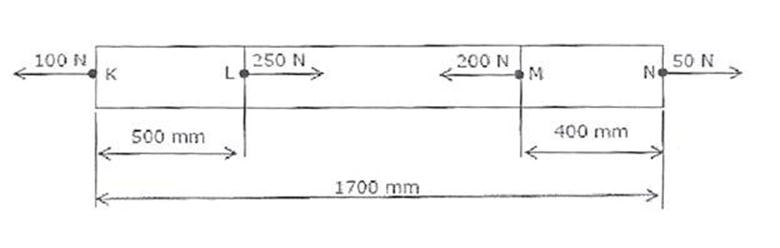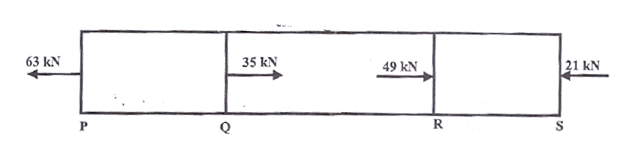# Stress and Strain

1. Select the proper sequence in an Engineering stress-strain curve diagram

1. Proportional Limit

2. Elastic limit

3. Yielding

4. Failure

(a) 2, 3, 1, 4

(b) 2, 1, 3, 4

(c) 1, 3, 2, 4

(d) 1, 2, 3, 4

Answer

2. Which one of the following materials is highly elastic?

(a) Rubber

(b) Brass

(c) Steel

(d) Glass

Answer

3. Assertion (A): Stress-strain curves for brittle material do not exhibit yield point.
Reason (R): Brittle materials fail without yielding.

(a) Both A and R are individually true and R is the correct explanation of A

(b) Both A and R are individually true but R is NOT the correct explanation of A

(c) A is true but R is false

(d) A is false but R is true

Answer

4. A steel bar of 40 mm × 40 mm square cross-section is subjected to an axial compressive load of 200 kN. If the length of the bar is 2 m and E = 200 GPa, the elongation of the bar will be:

(a) 1.25 mm

(b) 2.70 mm

(c) 4.05 mm

(d) 5.40 mm

Answer

5. Which of the following expresses the total elongation of a bar of length L with a constant cross-section of A and modulus of Elasticity E hanging vertically and subject to its own weight W?

(a)

(b)

(c)

(d)

Answer

6. The stretch in a steel rod of circular section, having a length ‘l’ subjected to a tensile load’ P’ and tapering uniformly from a diameter d1 at one end to a diameter d2 at the other end, is given

(a)

(b)

(c)

(d)

Answer

7. The ultimate tensile strength of a material is 400 MPa and the elongation up to maximum load is 35%. If the material obeys power law of hardening, then the true stress-true strain relation (stress in MPa) in the plastic deformation range is:

(a)

(b)

(c)

(d)

Answer

8. The deformation of a bar under its own weight as compared to that when subjected to a direct axial load equal to its own weight will be:

(a) The same

(b) One-fourth

(c) Half

(d) Double

Answer

9. Two identical circular rods of same diameter and same length are subjected to same magnitude of axial tensile force. One of the rods is made out of mild steel having the modulus of elasticity of 206 GPa. The other rod is made out of cast iron having the modulus of elasticity of 100 GPa. Assume both the materials to be homogeneous and isotropic and the axial force causes the same amount of uniform stress in both the rods. The stresses developed are within the proportional limit of the respective materials. Which of the following observations is correct?

(a) Both rods elongate by the same amount

(b) Mild steel rod elongates more than the cast iron rod

(c) Cast iron rod elongates more than the mild steel rod

(d) As the stresses are equal strains are also equal in both the rods

Answer

10. The figure below shows a steel rod of 25 mm2 cross sectional area. It is loaded at four points, K, L, M and N.Assume Esteel = 200 GPa. The total change in length of the rod due to loading is:

(a) 1 μm

(b) -10 μm

(c) 16 μm

(d) -20 μm

Answer

11. A bar having a cross-sectional area of 700mm2 is subjected to axial loads at the positions indicated. The value of stress in the segment QR is:(a) 40 MPa

(b) 50 MPa

(c) 70 MPa

(d) 120 MPa

Answer